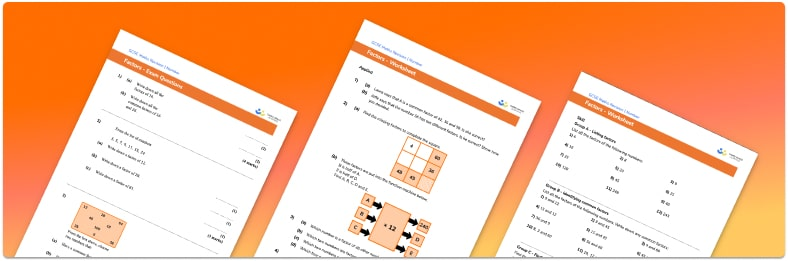# Factors worksheet• Section 1 of the factors worksheet contains 20+ skills-based factors questions, in 3 groups to support differentiation
• Section 2 contains 3 applied factors questions with a mix of word problems and deeper problem solving questions
• Section 3 contains 3 foundation and higher level GCSE exam style factors questions
• Answers and a mark scheme for all factors questions are provided
• Questions follow variation theory with plenty of opportunities for students to work independently at their own level
• All questions created by fully qualified expert secondary maths teachers
• Suitable for GCSE maths revision for AQA, OCR and Edexcel exam boards

• This field is for validation purposes and should be left unchanged.

You can unsubscribe at any time (each email we send will contain an easy way to unsubscribe). To find out more about how we use your data, see our privacy policy.

### Factors at a glance

The factors of a whole number are values which divide the number exactly. For example, the factors of 10 are 1, 2, 5 and 10. Factors are always integers. Prime numbers have exactly two factors, themselves and 1. Composite numbers have more than two factors.

The best way to find all the factors of a number is to identify factor pairs. Starting with 1, then 2 and 3 and so on, test whether the value is a factor of the given number. If it is, find the factor pair. Continue until you reach a value you already have as a factor.

For example, the factors of 24 are:

1 and 24

2 and 12

3 and 8

4 and 6

5 is not a factor and we already have 6 so we have all of the factors and we can stop. We could use the divisibility rules to test whether each value is a factor of the number.

The highest common factor of two numbers is the greatest value which is a factor of both numbers. The highest common factor can be found either by listing all the factors of each number or by considering the prime factors of each number and using a Venn diagram to identify the prime factors which are common to both numbers.

Looking forward, students can then progress to additional number worksheets, for example a multiplying and dividing decimals worksheet or an order of operations worksheet.For more teaching and learning support on Number our GCSE maths lessons provide step by step support for all GCSE maths concepts.

## Do you have KS4 students who need more focused attention to succeed at GCSE?There will be students in your class who require individual attention to help them succeed in their maths GCSEs. In a class of 30, it’s not always easy to provide.

Help your students feel confident with exam-style questions and the strategies they’ll need to answer them correctly with our dedicated GCSE maths revision programme.

Lessons are selected to provide support where each student needs it most, and specially-trained GCSE maths tutors adapt the pitch and pace of each lesson. This ensures a personalised revision programme that raises grades and boosts confidence.

Find out more